﻿ Area Formulas: Triangle, Parallelogram, Trapezoid
AREA FORMULAS: TRIANGLE, PARALLELOGRAM, TRAPEZOID
by Dr. Carol JVF Burns (website creator)
Follow along with the highlighted text while you listen!
• PRACTICE (online exercises and printable worksheets)

To begin this lesson, click the ‘Create a random triangle’ button below.
Then, repeatedly click the other button.

Do it again and again and again.
Make sure you understand what is meant by base/height pairs in a triangle.

The triangles are randomly-generated, so you might see some ‘unsatisfying’ situations, like really skinny triangles!

A precise discussion of base/height pairs follows this JSXGraph exploration.

EXPLORING BASE/HEIGHT PAIRS FOR A TRIANGLE

EVERY TRIANGLE HAS THREE BASE/HEIGHT PAIRS

Take a vertex of a triangle,
and drop a segment that is perpendicular to the opposite side (first picture below)
or (if needed) to an extension of the opposite side (second picture below).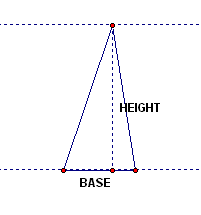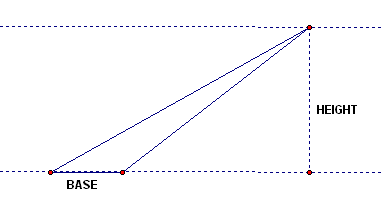The segment just created is called a height or altitude of the triangle,
and the side opposite this vertex is called the corresponding base.

Since every triangle has three vertices, every triangle has three base/height pairs .
If someone asks you for a ‘height and base of a triangle’ there are three possible correct answers!
(Depending on the shape and orientation of the triangle, however, one of these base/height pairs might be most obvious.)

The phrase ‘height (or base) of a triangle’ can refer to the actual line segment,
or to the length of the line segmentthe context will determine which is desired.

FINDING THE AREA OF A TRIANGLE
 Look at the picture at right, where the altitude (from top to bottom)has been cut in half. Convince yourself that the yellow triangles are congruent, and the grey triangles are congruent. (The AAS congruence theorem might be helpful.) This picture shows that the formula for the area of a triangle is half the height, times the base. (Why? Keep reading!) Here's how to ‘interpret’ the picture. Imagine the triangle is made of cardboard. Cutting it into pieces and re-arranging these pieces doesn't change the area. Cut off the ‘top’ grey piece and place it in the lower-left grey position. Cut off the ‘top’ yellow piece and place it in the lower-right yellow position. Now, the cardboard triangle has been re-arranged into a rectangle! What is the height of this rectangle? Half the height of the original triangle. What is the width of this rectangle? The base of the original triangle.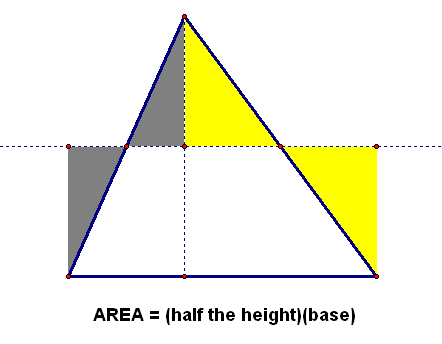AREA OF A TRIANGLE
Let $\,b\,$ and $\,h\,$ denote a base/height pair for a triangle.

The area of the triangle is given by the formula $\displaystyle\frac{1}{2}bh\,$.

Of course, this formula can be written in a variety of ways:
$$\cssId{s44}{\frac{1}{2}bh = \frac{1}{2}hb} \cssId{s45}{= \frac{bh}2} \cssId{s46}{= \frac{hb}2} \cssId{s47}{= \frac{h}{2}\cdot b} \cssId{s48}{= \frac{b}{2}\cdot h} \cssId{s49}{= \ldots}$$

With the formula for the area of a triangle in hand,
it is now easy to find area formulas for quadrilaterals that have at least one pair of parallel sides,
like parallelograms and trapezoids.

Whenever a quadrilateral has a pair of parallel sides,
then the (perpendicular) distance between these parallel sides is called the height or altitude,
and the parallel sides are called the bases.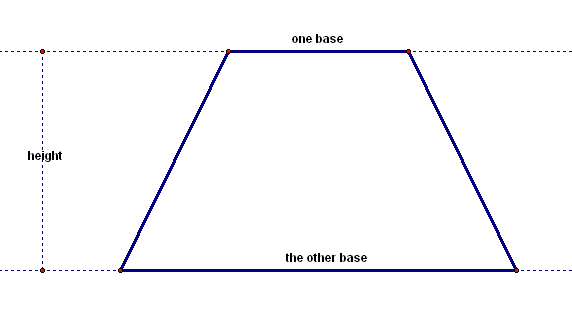Since a parallelogram has two pairs of parallel sides,
it has two base/height pairs: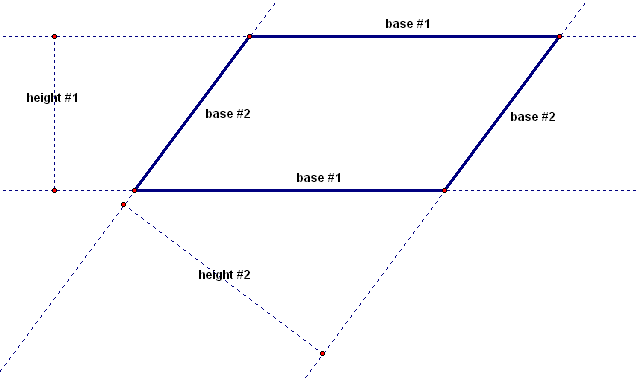The secret to finding the area of a parallelogram is to put in a diagonal,
and observe that the area is broken into two triangles!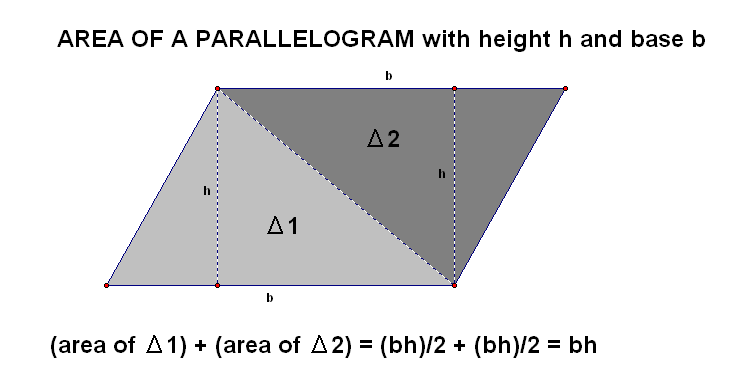AREA OF A PARALLELOGRAM
Let $\,b\,$ and $\,h\,$ denote a base/height pair for a parallelogram.

The area of the parallelogram is given by the formula $\,bh\,$.
Exactly the same idea is applied to find the area of a trapezoid: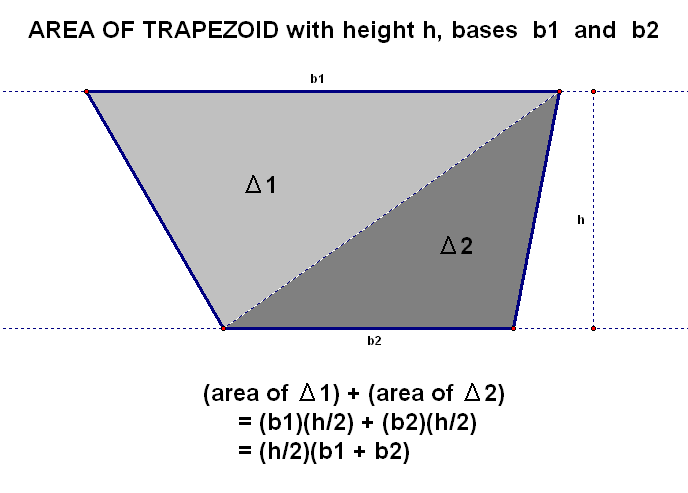AREA OF A TRAPEZOID
Let $\,h\,$ be the height of a trapezoid, and let $\,b_1\,$ and $\,b_2\,$ denote the two bases.

The area of the trapezoid is found by averaging the two bases, and multiplying by the height:
$$\cssId{s68}{\text{area} = h\left(\frac{b_1 + b_2}{2}\right)}$$
Of course, this formula can be written in a variety of ways:
$$\cssId{s70}{\text{area} = h\left(\frac{b_1 + b_2}{2}\right)} \cssId{s71}{= \left(\frac{b_1}2 + \frac{b_2}2\right)h} \cssId{s72}{= \frac{hb_1}2 + \frac{hb_2}2} \cssId{s73}{= \frac{1}{2}(b_1+b_2)h} \cssId{s74}{= \ldots}$$
Master the ideas from this section